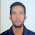## March 19, 2017

By:   Last Updated: in: ,

1.     Applied Numerical Methods BY Steven C. Chapra
Title of the book: Applied Numerical Methods
Authors: Steven C. Chapra
Contents:
Mathematical Modeling, Numerical Methods, and Problem Solving
MATLAB Fundamentals
Programming with MATLAB
Round off and Truncation Errors
Roots: Bracketing Methods
Roots: Open Methods
Optimization
Linear Systems
Linear Algebraic Equations and Matrices
Gauss Elimination
LU Factorization
Matrix Inverse and Condition
Iterative Methods
Eigenvalues
Linear Regression
General Linear Least-Squares and Nonlinear Regression
Fourier analysis
Polynomial Interpolation
Splines and Piecewise Interpolation
Numerical Integration Formulas
Numerical Integration of Functions
Numerical Differentiation
Initial-Value Problems
Boundary-Value Problems

2.   Multipysics Modeling using COMSOL by Roger W. Pryor
Title of the book:  Multipysics Modeling using COMSOL
Authors: Roger W. Pryor
Contents:
Modeling Methodology
Materials and Databases
1D Modeling
2D Modeling
2D Axisymmetric Modeling
2D Simple Mixed-Mode Modeling
2D Complex Mixed-Mode Modeling
3D Modeling
Perfectly Matched Layer Models
Bioheat Models
I will add new books in future so friends keep connect with us. If you find this post helpful please share with your friends and classmate and facebook whatsapp groups.
Also view  :-

Write comment
1.ohh grt..
thanx

2.Very Nice Post... Thanks For Sharing

3.good post.

4.5.